## Circular Motion - Complete Toolkit

### Objectives

1. To describe the direction of the velocity, acceleration, and net force for an object that moves in a circle at a constant speed.
2. To use the law of inertia to explain why a person moving in a circle experiences a sensation of being pushed outward and to identify reasons why the outward net force is a fictitious force.
3. To mathematically relate the speed, acceleration, radius, mass and net force for an object moving in a circle and to use such relationships to solve physics word problems.
4. To construct a free-body diagram for any given situation in which an object is moving in a circle.
5. To combine Newton’s second law of motion, free-body diagrams and circular motion equations to determine the value of the acceleration or an individual force for any situation involving an object moving in a circle.

### Interactive Simulations

1. Simple Circular Motion ModelGood model for beginners to explore the forces acting on a person riding a merry-go-round.  The 3D formatting is very useful for viewing the motion from a variety of perspectives.  By using sliders, students discover for themselves how rotational speed and radial distance interact to create a more thrilling ride.  Teachers:  the source code for this simulation is openly available, making this a customizable resource.

2. Merry Mixer Ride Model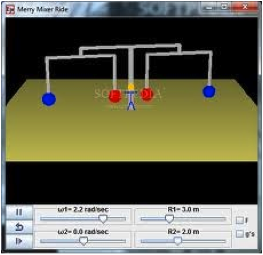Anyone who has ridden "The Scrambler" ride at an amusement park will be familiar with the system depicted in this simulation. It shows the interaction of two superimposed circular motions. The main frame of the ride rotates in one direction, while a second rotation at the ends of the frame arms carries the riders in an additional circular motion, usually in the opposite direction. Students can control the two rotation rates and the radii of the two orbits. Horizontal force vectors can be displayed, as well as a graph of the g-force on the rider vs. time.  Teachers: this model also provides open source access to the Java source code, making it a customizable resource.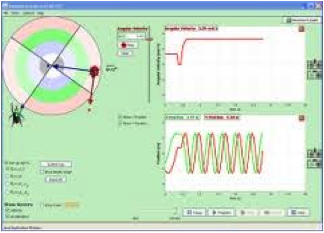Join the ladybug in an exploration of rotational motion. Rotate the merry-go-round to change its angle, or choose a constant angular velocity or angular acceleration. Explore how circular motion relates to the bug’s position, velocity, and acceleration using vectors or graphs.

Supplementary PhET Materials:  See link below for a short activity developed by a team of high school teachers to guide students in using the PhET simulation "Ladybug Revolution".  Students will produce data tables to promote understanding of the relationship among acceleration, velocity, and radius of the circular path. It also provides explicit guidance in exploring velocity and acceleration vectors. Includes lesson plan and student guide.  http://phet.colorado.edu/en/contributions/view/3234

4. The Physics Classroom: Uniform Circular Motion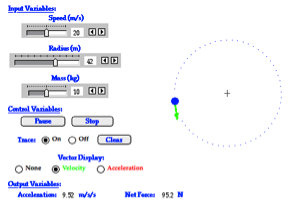This simulation allows the user to alter the radius and speed of an object moving in uniform circular motion to see the effect upon acceleration and force. The direction of the velocity and the force are displayed as vector arrows. This simulation is accompanied by an activity sheet with directions and exploration questions.

http://www.physicsclassroom.com/shwave/ucmdirns.cfm

### Video and Animations

1. PBS Learning Media: Centripetal Force in a Roller Coaster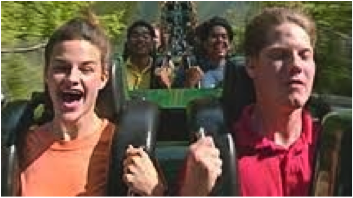This 5-minute video does a remarkably good job of explaining why you feel a sensation of being thrown outward from the center during circular motion, although there is no outward net force. It will help students differentiate centripetal force from the fictitious "centrifugal force".

2. Circular MotionThis learning cycle features 11 short videos by Rutgers Physics/Astronomy Education Research Group, organized sequentially for introducing circular motion in introductory physics courses. Each video includes learning goal, prior information needed to understand the material, and elicitation questions. Designed for cooperative learning, students will engage in thinking experiments, observation, and application. The materials were designed to mirror the activities of scientists when they construct and apply knowledge. Registered teacher-users have access to additional instructional guidance and resource material.

3. The Science of Speed:  TurningThis 5-minute video explores centripetal force from the context of NASCAR. How can tracks and race car tires be engineered to help drivers make faster turns? And how does the angle (bank) of the track affect centripetal acceleration (it makes a huge difference). Hear from engineers, physicists, and NASCAR drivers about the components that supply the centripetal force requirement for the race car to move around the curve.

4. Circus Physics:  Centripetal AccelerationThis video lesson uses horse trick riding to show students the basics of circular motion and centripetal acceleration. The video can serve to help students understand that an unbalanced force can change the direction of the velocity vector but not its magnitude. It includes tips on how to incorporate the video into instruction, discussion questions, and accompanying classroom activities. (Video is 2 minutes; allow about 25-30 minutes for complete activity.)

### Labs and Investigations

1. The Physics Classroom, The Laboratory, Making the Turn Lab

Students use simple materials (ball, board, wood block) to discover that an inward force is required for circular motion and without such a force and object continues along a straight-line path.

2. The Physics Classroom, The Laboratory, Loop the Loop Lab

Students use a bucket of water and a strong rope to gain a sense as to the relative strength of the tension force on a bucket when swung in a vertical circle; free-body diagrams and calculations are used to explain the observations.

3. The Physics Classroom, The Laboratory, Race Track Lab

Students use a computer simulation to explore the direction of forces required to successfully guide a car around an oval track and explain the principles associated with a successful strategy.

### Demonstration Ideas

1. Teaching from Space:  Centripetal ForceWatch a NASA astronaut onboard the International Space Station demonstrate centripetal force in a way that sheds a whole new light for students.  The video was shot in a weightless environment – the astronaut swings a tethered tool around a cord, rotates a bag of tea to show how air bubbles are pushed to the center, and spins a water droplet to show deformation resulting from centripetal force.

### Minds On Physics Internet Modules

The Minds On Physics Internet Modules are a collection of interactive questioning modules that target a student’s conceptual understanding. Each question is accompanied by detailed help that addresses the various components of the question.
1. Circular Motion and Gravitation, Ass’t CG1 -  Speed and Velocity
2. Circular Motion and Gravitation, Ass’t CG2 -  Acceleration and Net Force
3. Circular Motion and Gravitation, Ass’t CG3 -  Centripetal Force and Inertia
4. Circular Motion and Gravitation, Ass’t CG4 -  The Centripetal Force Requirement
5. Circular Motion and Gravitation, Ass’t CG5 -  Mathematical Analysis of Circular Motion

### Concept Building Exercises

1. The Curriculum Corner, Circular Motion, Speed and Velocity
2. The Curriculum Corner, Circular Motion, Acceleration and Circular Motion
3. The Curriculum Corner, Circular Motion, Circular Motion and Inertia
4. The Curriculum Corner, Circular Motion, The Centripetal Force Requirement
5. The Curriculum Corner, Circular Motion, Mathematics of Circular Motion

### Problem-Solving Exercises

1. The Calculator Pad, Circular Motion and Gravitation, Problems #1-15

### Science Reasoning Activities

1. Science Reasoning Center, Roller Coaster Loops

### Real Life Connections

1. Designing a Highway Exit Problem-Based Learning Module

http://pbl.ccdmd.qc.ca/resultat.php?action=clicFiche&he=1050&afficheRecherche=-1&IDFiche=141&endroitRetour=0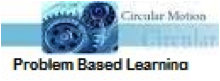A 2-day experiential learning activity for introductory physics relating to circular motion and friction.  In this scenario, students design a flat circular highway exit and determine, within a set of given constraints, what the exit speed limit should be. Students will apply concepts of rotational kinematics, static and kinetic friction, and will explore the dynamics involved in an object moving in a circular path. True to the PBL method, students will sift through information to separate useful from irrelevant data, locate missing information on their own, and then apply physics in finding solutions.### Common Misconceptions

1. Velocity, Force and the Inertial Path

An object that moves along a curved path experiences a velocity that is directed tangent to the path. At all points along a circle or curved path, the object’s velocity is directed straight ahead. So this tangent direction or straight ahead direction describes the inertial path of the object. If all the forces were balanced, then the object would continue along this inertial path. Movement in a circle is a deviation from this straight-ahead direction and thus requires an unbalanced force. Motion in a circle requires a net force directed towards the circle’s center in order to cause the object to deviate from the inertial path. But if this net inward force is removed at any instant, then the object travels along the inertial path tangent to the circle.

2. Acceleration

It is a very common belief among students that an object that is moving in a circle at a constant speed is not accelerating. But this is quite untrue. Moving in a circle involves an acceleration … even if it is a constant speed motion. Accelerating objects are changing their velocity. Being a vector, the velocity of an object describes an objects speed and direction. So objects that are changing either their speed or their direction are accelerating. As such, an object that moves in a circle at a constant speed is accelerating due to its change in direction. It is helpful to remind students what the three controls are on a car that allow it to accelerate – brake pedal for slowing down, gas pedal for speeding up, and steering wheel for changing directions.

3. The Notion of an Outward or Centrifugal Force

Anyone who has been on a roller coaster car, or a circular ride at an amusement park, or simply taken a sharp left-hand turn as a passenger in a car has experienced what seemed to be a strong push or force that is directed away from the center of the circle. This feelings of being pushed outward is undeniable; yet reality is not always consistent with one’s feelings. The sensation of an outward force is attributed to inertia – the tendency of an object to continue in motion in the same direction that it is heading when not acted upon by an unbalanced force. In these cases – roller coaster, amusement park ride, turning car – you are continuing in a straight line until your inertial path meets up with a car door or wall or some other obstacle. Upon meeting the car door or wall, you begin to push outward upon it. Meanwhile, the door or wall is pushing inward on you – consistent with Newton’s third law.

Many have written of these experiences by referring to the notion of a centrifugal or outward force. Those who have generally fall into two categories – those who don’t know what they are writing about and shouldn’t be writing about it and those who do know what they are writing about and must be cautious that they don’t mislead their readers. In the case of the latter, the discussion of a centrifugal force is almost always accompanied by some concluding remark that goes like this: “Such a force is a fictitious force. It does not exist despite the fact that the notion of it may help to explain the sensation of being pushed outward.” If the reader fails to observe the importance of this short phrase, then the reader is misled into believing that motion in a circle is accompanied by a centrifugal or outward force. That’s tragic.

### Elsewhere on the Web

1. Physlet Physics:  Rotations about a Fixed Axis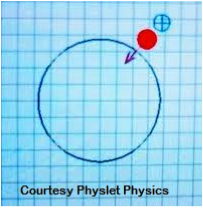This comprehensive set of animation-based problems is designed to help students understand the forces at play when an object rotates around a fixed axis (circular motion).  You’ll find 3 conceptual illustrations, mathematical calculations, explorations supplemented with student worksheets, and more than a dozen concept questions that could be flexibly used in cooperative groups or with full-class response systems (clickers).  Now freely available on the ComPADRE digital library.   (Appropriate for AP or Honors Physics)

2. Physlet Physics:  Circular Motion Animation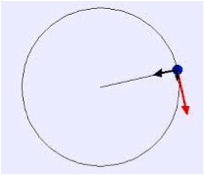This Physlet is intended as a warm-up question for the study of circular motion. It can be flexibly used as an animated demonstration, a concept question for student response systems, or as an exercise to elicit prior notions.### Standards

A. Next Generation Science Standards (NGSS) – High School

Performance Expectations
• HS-PS2.1:   Analyze data to support the claim that Newton’s second law of motion describes the mathematical relationship among the net force on a macroscopic object, its mass, and its acceleration.

Disciplinary Core Ideas
• HS-PS2.A.i:  Newton’s Second Law accurately predicts changes in the motion of macroscopic objects

Crosscutting Concepts
Crosscutting Concept #4: Systems and System Models
• Develop and use models (physical, mathematical, and computer models) to predict the behavior of a system and design systems to do specific tasks.

Science and Engineering Practices
Practice #2:  Developing and Using Models
• Use a model to provide mechanistic accounts of phenomena.

Practice #4: Analyzing and Interpreting Data
• Analyze data using tools, technologies, and/or models (e.g., computational, mathematical) in order to make valid and reliable scientific claims or determine an optimal design solution.
• Analyze data using computational models in order to make valid and reliable scientific claims.

Practice #5:  Using Mathematics and Computational Thinking
• Use mathematical representations of phenomena to describe explanations.
• Create a computational model or simulation of a phenomenon, designed device, process, or system.

Practice #6:  Designing Solutions (Engineering)
• Design, evaluate, and/or refine a solution to a real-world problem, based on scientific knowledge, student-generated sources of evidence, prioritized criteria, and tradeoff considerations.

Practice #8: Obtaining, Evaluating, and Communicating Information
• Communicate technical information or ideas (e.g. about phenomena and/or the process of development and the design and performance of a proposed process or system) in multiple formats (including orally, graphically, textually, and mathematically)

B. Common Core Standards for Mathematics (CC) – Grades 9-12

Standards for Mathematical Practice
• MP.1 – Make sense of problems and persevere in solving them
• MP.2 – Reason abstractly and quantitatively
• MP.4 – Model with mathematics

High School Algebra: Seeing Structure in Expressions
• A-SSE.1.a:     Interpret parts of an expression, such as terms, factors, and coefficients
• A-SSE.2:     Use the structure of an expression to identify ways to rewrite it.

High School Algebra:  Creating Equations
• A-CED.2    Create equations in two or more variables to represent relationships between quantities, graph equations on coordinate axes with labels and scales
• A-CED.4     Rearrange formulas to highlight a quantity of interest, using the same reasoning as in solving equations

High School Algebra:  Trigonometric Functions – High School
• F-TF.1     Understand radian measure of an angle as the length of the arc on the unit circle subtended by the angle

High School Algebra:  Linear, Quadratic, and Exponential Models – High School
• F-LE.5     Interpret the parameters in a linear or exponential function in terms of a context

High School Geometry:  Circles – High School
• G-C.4    Construct a tangent line from a point outside a given circle to the circle

C. Common Core Standards for English/Language Arts (ELA) – Grades 9-12

Reading Standards: Science and Technical Subjects – Key Ideas and Details
• RST.11-12.2    Determine the central ideas or conclusions of a text; summarize complex concepts, processes, or information presented in a text by paraphrasing them in simpler but still accurate terms.
• RST.11-12.3    Follow precisely a complex multistep procedure when carrying out experiments, taking measurements, or performing technical tasks; analyze the specific results based on explanations in the text.

Science and Technical Subjects – Integration of Knowledge and Ideas
• RST.11-12.9    Synthesize information from a range of sources (e.g., texts, experiments, simulations) into a coherent understanding of a process, phenomenon, or concept, resolving conflicting information when possible.

Science and Technical Subjects – Range of Reading and Level of Text Complexity
• RST.11-12.10     By the end of grade 12, read and comprehend science/technical texts in the grades 11-CCR text complexity band independently and proficiently.

D. College Ready Physics Standards (Heller and Stewart)

Standard 3:  Newton’s Laws of Motion – Grades 9-12

Objective 3.1:  Constant and Changing Motion
• H-3.1.1 – The displacement, or change in position, of an object is a vector quantity that can be calculated by subtracting the initial position from the final position, where initial and final positions can have positive and negative values. Displacement is not always equal to the distance traveled.

Learning Outcome:
• Translate between different representations of the motion of objects: verbal and/or written descriptions, motion diagrams, data tables, graphical representations (position versus time graphs and instantaneous velocity versus time graphs) and mathematical representations.

Objective 3.2:  Forces and Change in Motion – Middle School
• Objective M-3.2.2d – A force of constant magnitude acting at right angles to the direction of the object’s motion causes the object to move in a circle at a constant speed.
• Objective M-3.2.3 – The forces acting on an object can be represented by arrows (vectors) drawn on an isolated picture of the object, called a force diagram. The direction of each arrow shows the direction of the push or pull. Forces are labeled: “(type of interaction) push or pull of (interacting object) on the (object of interest).

Objective 3.2:  Forces and Change in Motion – High School
• Objective H.3.2.2c – An object moves in a circle when the vector sums of all the forces (net force) is constant in magnitude, always directed at right angles to the direction of motion and always directed toward the same point in space, the center of the circle. The speed of the object does not change; the acceleration causes the continual change in the direction of the change-in-velocity vector.

Learning Outcomes:
• Analyze force diagrams to determine if they accurately represent different situations involving multiple contact, gravitational and/or electrical interactions. When appropriate, determine the one-dimensional vector sum of all the forces (net force), and interpret the meaning of the vector sum of all the forces (net force).
• Represent the forces acting on the object of interest by drawing a force diagram showing both the vertical and horizontal forces. When appropriate, use vector addition to determine the relative size and direction of the sum of all the forces (net force), and interpret the meaning of the net force.
• Investigate and explain why an object moving at a constant speed in a circle is accelerating. Justify the explanation by constructing a motion diagram and by using knowledge of acceleration and Newton’s second law.